# RBI Assistant Pre 2023 Reasoning Ability Quiz –5

## RBI Assistant Pre 2023 Reasoning Ability Quiz

Almost all major competitive exams in the country have a section on Reasoning Ability, and it is also one of the most scoring sections. Candidates can ace this section in exams if they practice regularly in a dedicated manner. So here we are providing you with the RBI Assistant Pre 2023 Reasoning Ability Quiz to help you prepare better. This RBI Assistant Pre 2023 Reasoning Ability Quiz includes all of the most recent pattern-based questions, as well as Previous Year Questions. This RBI Assistant Pre 2023 Reasoning Ability Quiz is totally FREE. Candidates will be provided with a detailed explanation of each question in this RBI Assistant Pre 2023 Reasoning Ability Quiz. Candidates must practice this RBI Assistant Pre 2023 Reasoning Ability Quiz to achieve a good score in the Reasoning Ability Section.

Directions (1-5): In each of the questions given below three statements are followed by some conclusions. You have to take the given statements to be true even if they seem to be at variance from commonly known facts. Read all the conclusions and then decide which of the given conclusions logically follows from the given statements disregarding commonly known facts.

1. Statements: Some abacus is book

No book is card

Some card is desk

Conclusions:       I. Some desk can never be book

II. No abacus is card.

III. All desk are book.

(a)Only I follow

(b)Only II follow

(c)Only III follow

(d)All follow

(e)None of the above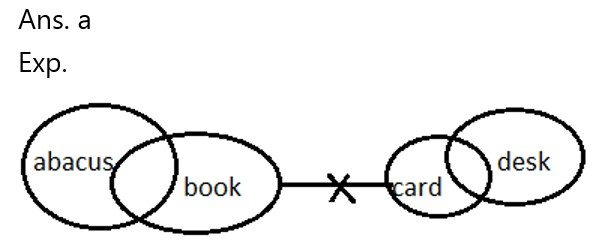1. Statements: No sea is river

No river is lake

No lake is ocean

Conclusions:      I. No sea is lake

II. No river is ocean

III. Some river is ocean

(a) Only I and III follow

(b) either II or III follow

(c) either I or II  follow

(d)None follows

(e)All follows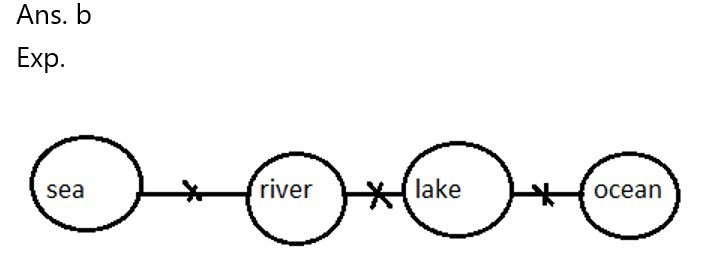1. Statements: I. All pants are top

II.All tops are shirts

III. No top is coat

Conclusions:       I. Some coat can never be pants

II. Only shirts are pants

III. No shirt is coat

(a)Only I and IV follow

(b)Only I and II follow

(c)Only III and IV follow

(d) Only I follow.

(e) None of these.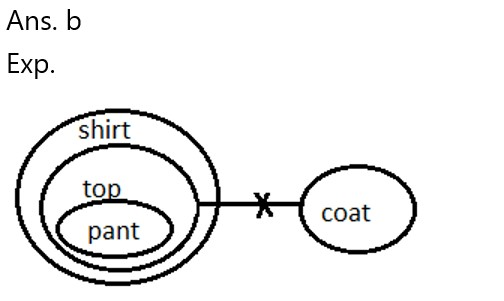1. Statements: Some month is day

Some days is week

All day is year

Conclusions:       I. No week is month

II. some week can be month

III. No year is month

(a) Only I and III follow

(b) either II or III follow

(c) either I or II  follow

(d) All follows

(e) None of these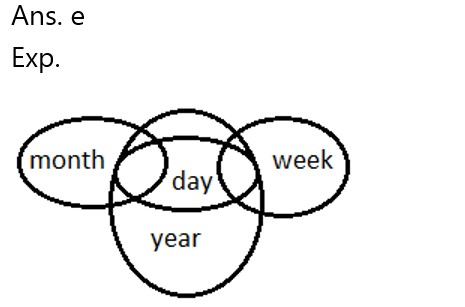1. Statements:   I. No red is blue

II.Some blue is black

III. All black is white

Conclusions:        I. Some red can never be blue

II.  Some black can never be red

III. No blue is white

(a)Only I and IV follow

(b)Only I and II follow

(c)Only III and IV follow

(d)Only I follows.

(e)None of these .Directions (6-10): Each of the questions below consists of question and two statements numbered I, and II given below it. You have to decide whether the data provided in the statements are sufficient to answer the question. Read both the statements and give answer.

1. There are six friends i.e. A, B, C, D, E and F, who is the smallest in height?

I. B is taller than C and D but smaller than A. E is taller than A, but not the tallest.

II. F is taller than E and B. C is smaller than D.

(a) If the data in statement I alone are sufficient to answer the question while the data in statement II are not sufficient to answer the question.

(b) If the data in statement II alone are sufficient to answer the question while the data in statement I are not sufficient to answer the question.

(c)  If the data either in statement I alone or in statement II alone are sufficient to answer the question.

(d) If the data in both statement I and statement II are not sufficient to answer the question.

(e) If the data in both statement I and statement II are sufficient to answer the question.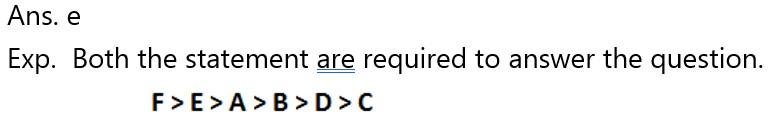1. Who among the following five i.e. P, Q, R, S and T is sitting on the middle of the row (all are facing north direction)?

I. S sit 2nd to the left of T and on the immediate right of Q. T sits on the immediate left of R, who sits on the right end.

II. P sits 2nd to the right of R who sits on left end of the Row. S is not the immediate neighbor of P.

(a) If the data in statement I alone are sufficient to answer the question while the data in statement II are not sufficient to answer the question.

(b) If the data in statement II alone are sufficient to answer the question while the data in statement I are not sufficient to answer the question.

(c)  If the data either in statement I alone or in statement II alone are sufficient to answer the question.

(d) If the data in both statement I and statement II are not sufficient to answer the question.

(e) If the data in both statement I and statement II are sufficient to answer the question.1. On which day does Rahul celebrate his birthday if his brother and mother both are right?

I. Rahul’s brother remembers that his birthday is before 25th but after 22nd of November.

II. Rahul’s mother remembers that his birthday is after 21st but before 24th of November.

(a) If the data in statement I alone are sufficient to answer the question while the data in statement II are not sufficient to answer the question.

(b) If the data in statement II alone are sufficient to answer the question while the data in statement I are not sufficient to answer the question.

(c)  If the data either in statement I alone or in statement II alone are sufficient to answer the question.

(d) If the data in both statement I and statement II are not sufficient to answer the question.

(e) If the data in both statement I and statement II are sufficient to answer the question.

Ans. e

Exp.  Both the statement are required to answer the question i.e. Birthday is on 23rd November.

1. How is A related to H?

I. A is married to B. H is brother of F and the son of B .

II. B is Father of H. A is the child of E, who is married to B.

(a) If the data in statement I alone are sufficient to answer the question while the data in statement II are not sufficient to answer the question.

(b) If the data in statement II alone are sufficient to answer the question while the data in statement I are not sufficient to answer the question.

(c)  If the data either in statement I alone or in statement II alone are sufficient to answer the question.

(d) If the data in both statement I and statement II are not sufficient to answer the question.

(e) If the data in both statement I and statement II are sufficient to answer the question.

Ans. e

Exp.  Both the statement are required to answer the question i.e. Birthday is on 23rd November.

1. In which direction Rahul is with respect to Raman?

I. Rahul is facing north and Raman is facing east and both are standing in front of Raman’s house. Raman and Rahul started walking in their respective directions and both walks for 5km.

II. Rahul is to the south of Amaan. Raman is to the north of Shikha, who is 2km away from Amaan.

(a) If the data in statement I alone are sufficient to answer the question while the data in statement II are not sufficient to answer the question.

(b) If the data in statement II alone are sufficient to answer the question while the data in statement I are not sufficient to answer the question.

(c)  If the data either in statement I alone or in statement II alone are sufficient to answer the question.

(d) If the data in both statement I and statement II are not sufficient to answer the question.

(e) If the data in both statement I and statement II are sufficient to answer the question.